# Converting a String Variable to an Integer Variable or Double Variable in UiPathIn many automation processes, the numbers obtained from the screen need subsequent calculation. It is necessary for a UiPath developer to master how to convert a string variable to an integer variable or double variable in UiPath.

First of all, you may wonder why we need to do that. For example, there are two string variables, "2" and "3". When we add them together via a plus sign in expression, the expected result is "5". However, because they are string variables, the result will be "23".

So, if you want two string variables to add up as an integer (not string), you should convert them to an integer variable before adding them up.

In general, there are three methods to convert a string variable to an integer variable.

#### CInt, Convert.ToInt32, and Integer.Parse.

Follow these steps to use each method:

Step 1: Create two string variables and set their default value to "2" and 3"

Step 2: Add three Write Line activities to use those methods respectively

Step 3: Because of the Write Line activities you need a string type input argument, so we need to use ToString method to convert these results back to string type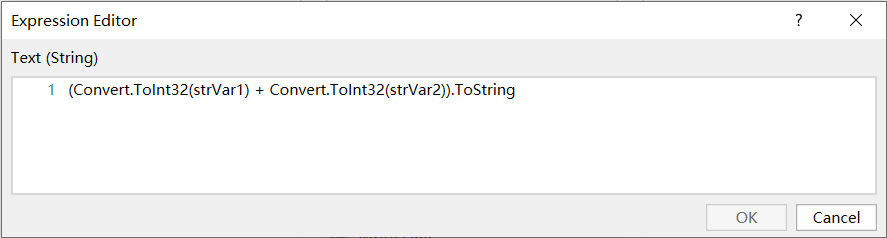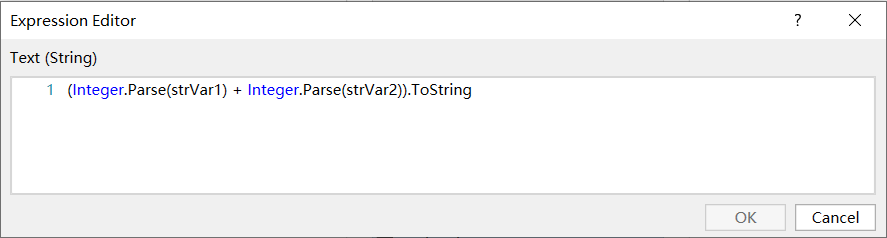If all the results are "5", then evidently all three of the methods have worked.

There are some differences between the three methods when an input string is not a correct integer format. If your string variable is not a number format such as "10a" then all of them will throw an exception.

But if the string is a number format that includes a decimal point such as "10.6" or "-8.2".

Convert.ToInt32 and Integer.Parse still throw an exception, but the CInt will return the rounded result "11" and "8". In that case,the result of the addition will be "3".

By the way, if the fractional component is halfway between two integers, one of which is even and the other odd, then the even number will be returned.

Because the integer variable just can retain the integer part of number information if we need to retain a fractional part, there are also three methods that can be used to convert a string variable to a double variable.

#### CDbl, Convert.ToDouble, and Double.Parse.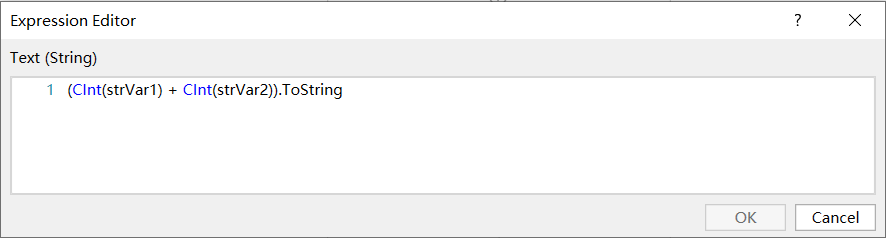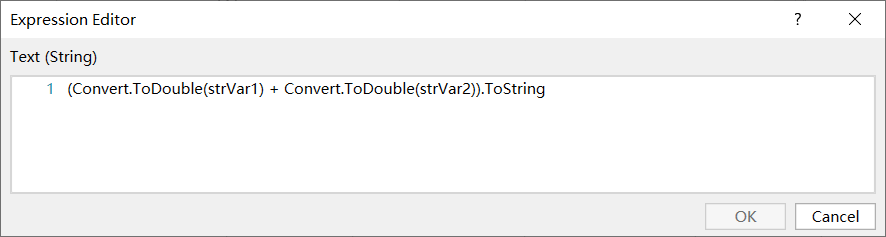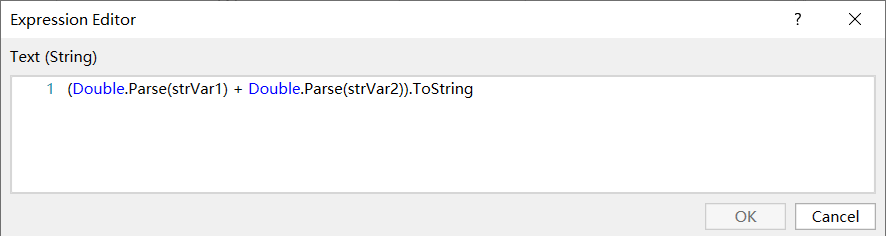If two string variables’ default values are "10.6" and “-8.4", then all the results will be "2.4".

Now, you will able to convert string variables to numeric variables for arithmetic operations, and you can also convert them back to string variables by using the ToString method.SiYang Wu

UiPath Community MVP and the RPA project director, NRI in Beijing, China

###### Events

UiPath is a registered trademark in the United States and several countries across the globe. See TMEP 906.